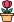엔지니어 게시판
LeetCode 솔루션 분류

# [11/27] 446. Arithmetic Slices II - Subsequence

### 작성자 정보

•작성
• 작성일

• 182 조회
• 1 댓글
• 0 추천
• 0 비추천

### 본문

Given an integer array `nums`, return the number of all the arithmetic subsequences of `nums`.

A sequence of numbers is called arithmetic if it consists of at least three elements and if the difference between any two consecutive elements is the same.

• For example, `[1, 3, 5, 7, 9]``[7, 7, 7, 7]`, and `[3, -1, -5, -9]` are arithmetic sequences.
• For example, `[1, 1, 2, 5, 7]` is not an arithmetic sequence.

subsequence of an array is a sequence that can be formed by removing some elements (possibly none) of the array.

• For example, `[2,5,10]` is a subsequence of `[1,2,1,2,4,1,5,10]`.

The test cases are generated so that the answer fits in 32-bit integer.

Example 1:

```Input: nums = [2,4,6,8,10]
Output: 7
Explanation: All arithmetic subsequence slices are:
[2,4,6]
[4,6,8]
[6,8,10]
[2,4,6,8]
[4,6,8,10]
[2,4,6,8,10]
[2,6,10]
```

Example 2:

```Input: nums = [7,7,7,7,7]
Output: 16
Explanation: Any subsequence of this array is arithmetic.
```

Constraints:

• `1  <= nums.length <= 1000`
• `-231 <= nums[i] <= 231 - 1`
Accepted
49,066
Submissions
120,562
태그

댓글 1

## 학부유학생님의 댓글

• 익명
• 작성일
Runtime: 3267 ms, faster than 24.85% of Python3 online submissions for Arithmetic Slices II - Subsequence.
Memory Usage: 185.7 MB, less than 14.20% of Python3 online submissions for Arithmetic Slices II - Subsequence.
``````from collections import defaultdict
class Solution:
def numberOfArithmeticSlices(self, nums: List[int]) -> int:
dp = defaultdict(int)
res = 0

for e in range(1, len(nums)):
for s in range(e):
diff = nums[e] - nums[s]
res += dp[(s, diff)]
dp[(e, diff)] += dp[(s,diff)] + 1

return res``````
전체 349 / 1 페이지

• 등록일 00:48
• 등록일 02.01
• 등록일 02.01
• 등록일 02.01
• 등록일 02.01
• 등록일 02.01
• 등록일 02.01
• 등록일 02.01
• 등록일 02.01
• 등록일 02.01
• 등록일 02.01
• 등록일 02.01

• 등록일 01.31
• 등록일 01.31
• 등록일 01.31
• 등록일 01.27
• 등록일 01.27
• 등록일 01.23
• 등록일 01.23

### Stats

• 현재 접속자 54 명
• 오늘 방문자 156 명
• 어제 방문자 1,716 명
• 최대 방문자 2,853 명
• 전체 회원수 535 명